Test: Class 10 Mathematics: CBSE Sample Question Paper- Term I (2021-22)

# Test: Class 10 Mathematics: CBSE Sample Question Paper- Term I (2021-22)

Test Description

## 50 Questions MCQ Test CBSE Sample Papers For Class 10 | Test: Class 10 Mathematics: CBSE Sample Question Paper- Term I (2021-22)

Test: Class 10 Mathematics: CBSE Sample Question Paper- Term I (2021-22) for Class 10 2022 is part of CBSE Sample Papers For Class 10 preparation. The Test: Class 10 Mathematics: CBSE Sample Question Paper- Term I (2021-22) questions and answers have been prepared according to the Class 10 exam syllabus.The Test: Class 10 Mathematics: CBSE Sample Question Paper- Term I (2021-22) MCQs are made for Class 10 2022 Exam. Find important definitions, questions, notes, meanings, examples, exercises, MCQs and online tests for Test: Class 10 Mathematics: CBSE Sample Question Paper- Term I (2021-22) below.
Solutions of Test: Class 10 Mathematics: CBSE Sample Question Paper- Term I (2021-22) questions in English are available as part of our CBSE Sample Papers For Class 10 for Class 10 & Test: Class 10 Mathematics: CBSE Sample Question Paper- Term I (2021-22) solutions in Hindi for CBSE Sample Papers For Class 10 course. Download more important topics, notes, lectures and mock test series for Class 10 Exam by signing up for free. Attempt Test: Class 10 Mathematics: CBSE Sample Question Paper- Term I (2021-22) | 50 questions in 90 minutes | Mock test for Class 10 preparation | Free important questions MCQ to study CBSE Sample Papers For Class 10 for Class 10 Exam | Download free PDF with solutions
 1 Crore+ students have signed up on EduRev. Have you?
Test: Class 10 Mathematics: CBSE Sample Question Paper- Term I (2021-22) - Question 1

### The product of two numbers is 1900 and their LCM is 100, then the HCF of the numbers is:

Detailed Solution for Test: Class 10 Mathematics: CBSE Sample Question Paper- Term I (2021-22) - Question 1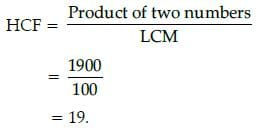Test: Class 10 Mathematics: CBSE Sample Question Paper- Term I (2021-22) - Question 2

### If a number when divided by 71 gives 37 as quotient and 42 as remainder, then the number is:

Detailed Solution for Test: Class 10 Mathematics: CBSE Sample Question Paper- Term I (2021-22) - Question 2

Here, divisor = 71, quotient = 37 and remainder = 42.
∴ Dividend = Divisor × Quotient + Remainder
= (71 × 37) + 42
= 2669.

Test: Class 10 Mathematics: CBSE Sample Question Paper- Term I (2021-22) - Question 3

### The decimal expansion of the rational number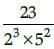will terminate after:

Detailed Solution for Test: Class 10 Mathematics: CBSE Sample Question Paper- Term I (2021-22) - Question 3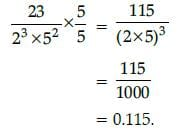Test: Class 10 Mathematics: CBSE Sample Question Paper- Term I (2021-22) - Question 4

The number 0.121212 ...... is the form of p/q will be equal:

Detailed Solution for Test: Class 10 Mathematics: CBSE Sample Question Paper- Term I (2021-22) - Question 4

Let x =0.121212.... ...(i)
100x =12.1212.... ...(ii)
On subtracting eqn. (i) from (ii)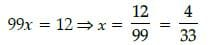Test: Class 10 Mathematics: CBSE Sample Question Paper- Term I (2021-22) - Question 5

If the sum of the zeroes of the quadratic polynomial 5x2 – px + 8 is 4, then the value of k is:

Detailed Solution for Test: Class 10 Mathematics: CBSE Sample Question Paper- Term I (2021-22) - Question 5

Let the zeroes of the given polynomial be a and b, then sum of the zeroes = 4
i.e  p/5  = 4
⇒  p = 20

Test: Class 10 Mathematics: CBSE Sample Question Paper- Term I (2021-22) - Question 6

If the polynomial is p(x) = x3 - 3x2 + x + 1, then the value of p(- 3) is:

Detailed Solution for Test: Class 10 Mathematics: CBSE Sample Question Paper- Term I (2021-22) - Question 6

We have,
p(x) = x3 – 3x2 + x + 1
Then, p(– 3) = (-3)3 – 3(– 3)2 + (– 3)
+ 1
= – 27 – 27 – 3 + 1
= – 56.

Test: Class 10 Mathematics: CBSE Sample Question Paper- Term I (2021-22) - Question 7

If α, β and γ are the zeroes of the polynomial 2x3 + x2 – 13x + 6, then abg is equal to:

Detailed Solution for Test: Class 10 Mathematics: CBSE Sample Question Paper- Term I (2021-22) - Question 7

Let the polynomial be f(x), then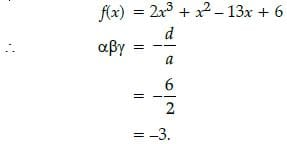Test: Class 10 Mathematics: CBSE Sample Question Paper- Term I (2021-22) - Question 8

The distance of the point P(– 4, 3) from the origin is:

Detailed Solution for Test: Class 10 Mathematics: CBSE Sample Question Paper- Term I (2021-22) - Question 8

Here, two points (–4, 3) and
(0, 0) are given, then
x1 = – 4, y1 = 3 and x2 = 0, y2 = 0
∴ Distance between them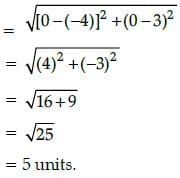Test: Class 10 Mathematics: CBSE Sample Question Paper- Term I (2021-22) - Question 9

If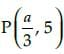is the mid point of the line segment joining the points A(–7, 3) and B(– 3, 7), then the value of a is:

Detailed Solution for Test: Class 10 Mathematics: CBSE Sample Question Paper- Term I (2021-22) - Question 9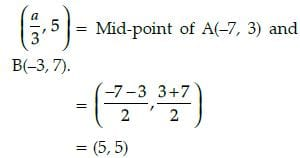Then, comparing on both sides, we get
a/3 = – 5
⇒ a = – 15.

Test: Class 10 Mathematics: CBSE Sample Question Paper- Term I (2021-22) - Question 10

The point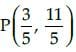divide the line segment joining the points A(3, 5) and (– 3, – 2) is the ratio:

Detailed Solution for Test: Class 10 Mathematics: CBSE Sample Question Paper- Term I (2021-22) - Question 10

Let the required ratio be k : 1, then the coordinates of P are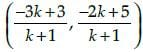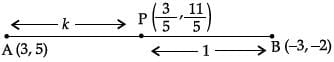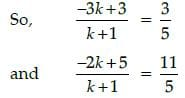⇒ –15k + 15 = 3k + 3 and –10k + 25 = 11k + 11
⇒ –18k = –12 and –21k = –14
⇒ k = 2/3  and k = 2/3
Hence the point P divides AB in the ratio
2 : 3.

Test: Class 10 Mathematics: CBSE Sample Question Paper- Term I (2021-22) - Question 11

The distance between the points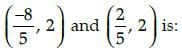Detailed Solution for Test: Class 10 Mathematics: CBSE Sample Question Paper- Term I (2021-22) - Question 11

Distance between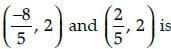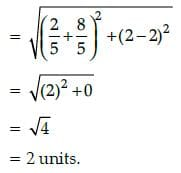Test: Class 10 Mathematics: CBSE Sample Question Paper- Term I (2021-22) - Question 12

In a given ABC, DE || BC and AB/DB = 3/5. If AC = 5.6 cm, then AE = .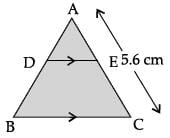Detailed Solution for Test: Class 10 Mathematics: CBSE Sample Question Paper- Term I (2021-22) - Question 12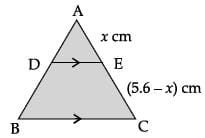Let AE = x cm,
then EC = (5.6 – x) cm
and DE || BC   (given)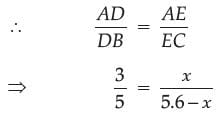⇒ 3(5.6 – x) = 5x
⇒ 16.8 – 3x = 5x
⇒ 8x = 16.8
⇒ x = 2.1

Test: Class 10 Mathematics: CBSE Sample Question Paper- Term I (2021-22) - Question 13

If sin A = 1/√2, then the value of tan A + cot A is:

Detailed Solution for Test: Class 10 Mathematics: CBSE Sample Question Paper- Term I (2021-22) - Question 13

∵ sin A = 1/√2,
∴ sin A = sin 45°
⇒ A = 45°
Now, tan A + cot A = tan 45° + cot 45°
= 1 + 1
= 2.

Test: Class 10 Mathematics: CBSE Sample Question Paper- Term I (2021-22) - Question 14

If x = 0, then the value of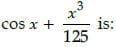Detailed Solution for Test: Class 10 Mathematics: CBSE Sample Question Paper- Term I (2021-22) - Question 14

We have,
x = 0
or cos x = cos 0
or cos x = 1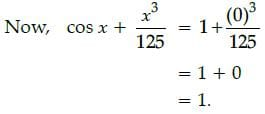Test: Class 10 Mathematics: CBSE Sample Question Paper- Term I (2021-22) - Question 15

The value of sin2 30° – cos2 30° is:

Detailed Solution for Test: Class 10 Mathematics: CBSE Sample Question Paper- Term I (2021-22) - Question 15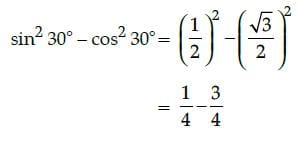= - 2/4
= - 1/2.

Test: Class 10 Mathematics: CBSE Sample Question Paper- Term I (2021-22) - Question 16

The circumference of a circle of radius 4 cm is: (Use π = 3.14)

Detailed Solution for Test: Class 10 Mathematics: CBSE Sample Question Paper- Term I (2021-22) - Question 16

Given, radius (r) = 4 cm
∴ Circumference, (C) = 2πr
= 2 × 3.14 × 4 cm
= 25. 12 cm.

Test: Class 10 Mathematics: CBSE Sample Question Paper- Term I (2021-22) - Question 17

Aarti had bought a circular pot wherein area of cross-section is mentioned as 38.5 cm2. She wantedto calculate the radius of the circle and observed its value.

Detailed Solution for Test: Class 10 Mathematics: CBSE Sample Question Paper- Term I (2021-22) - Question 17

Area of a circle = 38.5 cm2

πr2 = 38.5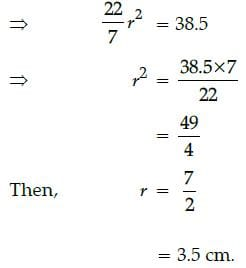Test: Class 10 Mathematics: CBSE Sample Question Paper- Term I (2021-22) - Question 18

If a piece of wire 30 cm long is bent into the form of an arc of a circle, subtending an angle of 60° at its centre, then radius of the circle is :

Detailed Solution for Test: Class 10 Mathematics: CBSE Sample Question Paper- Term I (2021-22) - Question 18

Length of arc = 30 cm
So, 2 x 5 – 5p + 2 = 0
⇒ 10 + 2 – 5p = 0
⇒ 5p = 12
⇒ p = 2.4

Test: Class 10 Mathematics: CBSE Sample Question Paper- Term I (2021-22) - Question 19

Which of the following can not be the probability of an event ?

Detailed Solution for Test: Class 10 Mathematics: CBSE Sample Question Paper- Term I (2021-22) - Question 19

Probability of an event can not be more than one or negative 9/8 > 1.

Test: Class 10 Mathematics: CBSE Sample Question Paper- Term I (2021-22) - Question 20

A box contains 80 discs, numbered from 1 to 90. If one disc is drawn at random from the box, the probability that it bears a prime number less than 30, is:

Detailed Solution for Test: Class 10 Mathematics: CBSE Sample Question Paper- Term I (2021-22) - Question 20

Total number outcomes = 80
i.e., n(S) = 80
Prime number less than 30 = 2, 3, 5, 7, 11,
13, 17, 19, 23, 29
i.e., n(E) = 10
∴ Required probability,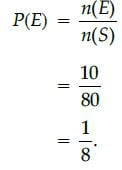Test: Class 10 Mathematics: CBSE Sample Question Paper- Term I (2021-22) - Question 21

What number must be added to numerator and denominator of 2/7 to make fraction equal to 2/3 ?

Detailed Solution for Test: Class 10 Mathematics: CBSE Sample Question Paper- Term I (2021-22) - Question 21

Let x be added to numerator and denominator of 2/7, so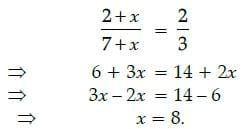Test: Class 10 Mathematics: CBSE Sample Question Paper- Term I (2021-22) - Question 22

The difference of LCM and HCF of 28 and 42 is:

Detailed Solution for Test: Class 10 Mathematics: CBSE Sample Question Paper- Term I (2021-22) - Question 22

28 = 22 × 7
and 42 = 2 x 3 x 7
∴ HCF (28, 42) = 2 x 7 = 14
and LCM (28, 42) = 22 x 3 x 7 = 84
Now, difference = 84 – 14 = 70.

Test: Class 10 Mathematics: CBSE Sample Question Paper- Term I (2021-22) - Question 23

If the sum of ages of father and son is 40 years and its difference is 20 years then age of father will be:

Detailed Solution for Test: Class 10 Mathematics: CBSE Sample Question Paper- Term I (2021-22) - Question 23

Let father's age be x years and son's age be y, then
x + y = 40 ...(i)
and x – y = 20
On adding eq. (i) and (ii), we get
2x = 60
⇒ x = 30.

Test: Class 10 Mathematics: CBSE Sample Question Paper- Term I (2021-22) - Question 24

If 2 sin 5θ = √3, then the value of q is:

Detailed Solution for Test: Class 10 Mathematics: CBSE Sample Question Paper- Term I (2021-22) - Question 24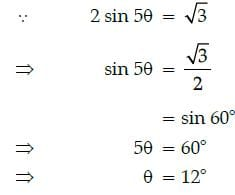Test: Class 10 Mathematics: CBSE Sample Question Paper- Term I (2021-22) - Question 25

The system of equations 2x + ay = 1 and 5x – 7y = 9 are given.
If the system has a unique solution, then all real values except :

Detailed Solution for Test: Class 10 Mathematics: CBSE Sample Question Paper- Term I (2021-22) - Question 25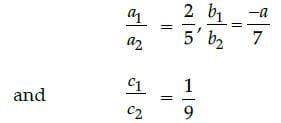∵ The system of equations has unique
solution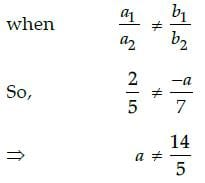i.e., all real values except - 14/5

Test: Class 10 Mathematics: CBSE Sample Question Paper- Term I (2021-22) - Question 26

If the radius of a circle with centre O is 3.5 cm and two radii OA and OB are drawn at right angles toeach other, then the area of minor segment is :

Detailed Solution for Test: Class 10 Mathematics: CBSE Sample Question Paper- Term I (2021-22) - Question 26

Here,
r = 3.5 cm, θ = 90°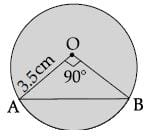∴ Area of minor segment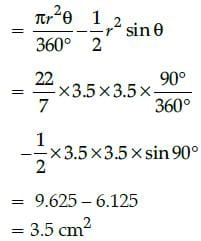Test: Class 10 Mathematics: CBSE Sample Question Paper- Term I (2021-22) - Question 27

If the area of a sector of the circle of radius 18 cm is 72π cm2, then the angle q is :

Detailed Solution for Test: Class 10 Mathematics: CBSE Sample Question Paper- Term I (2021-22) - Question 27

Here, r = 18 cm and area of sector = 72 π
cm2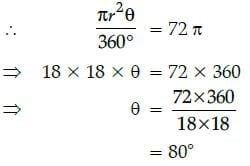Test: Class 10 Mathematics: CBSE Sample Question Paper- Term I (2021-22) - Question 28

The zeroes of the quadratic polynomial 3x2 + 2x – 1 = 0 are :

Detailed Solution for Test: Class 10 Mathematics: CBSE Sample Question Paper- Term I (2021-22) - Question 28

Given, 3x2 + 2x – 1 = 0
⇒ 3x2 + 3x – x – 1 = 0
⇒ 3x(x + 1) – 1 (x + 1) = 0
⇒ (x + 1) (3x – 1) = 0
⇒ x = – 1 and 1/3

Test: Class 10 Mathematics: CBSE Sample Question Paper- Term I (2021-22) - Question 29

In the given figure, if PQ = 8 cm and PR = 6 cm, then the radius of the circle is ..... cm, where O is thecentre of circle.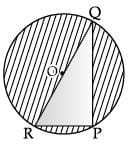Detailed Solution for Test: Class 10 Mathematics: CBSE Sample Question Paper- Term I (2021-22) - Question 29

Since ∠P in a semicircle, so
∠P = 90°
∴ In DRPQ, RQ2 = PR2 + PQ2
(Using Pythagoras theorem)
= (6)2 + (8)2
= 100
∴ RQ = 10 cm
∴ Radius = 1/2RQ
= 5 cm

Test: Class 10 Mathematics: CBSE Sample Question Paper- Term I (2021-22) - Question 30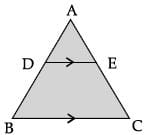In the given figure, If DE || BC, AD = 2 cm, BD = 2.5 cm, AE = 3.2 cm and DE = 4 cm, then BC = 9 cmFind AC.

Detailed Solution for Test: Class 10 Mathematics: CBSE Sample Question Paper- Term I (2021-22) - Question 30

∵ DE || BC (given)
∴ ∠ADE = ∠ABC (corresponding angles)
and ∠AED = ∠ACB (corresponding angles)
∴ DADE ~ DABC (By AA similarity)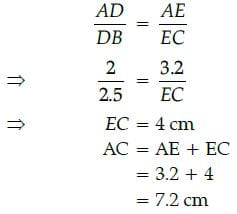Test: Class 10 Mathematics: CBSE Sample Question Paper- Term I (2021-22) - Question 31

If cot (A + B – C) = √3 and cos (B + C – A) = 1/2, then the value of B is :

Detailed Solution for Test: Class 10 Mathematics: CBSE Sample Question Paper- Term I (2021-22) - Question 31

cot (A + B + C) = √3 = cot 30°
⇒ A + B – C = 30° ...(i)
and cos (B + C – A) = 1/2
⇒ cos (B + C – A)= cos 60°
⇒ B + C – A = 60° ...(ii)
Adding eq. (i) and (ii), we get
2B = 90°
⇒ B = 45°

Test: Class 10 Mathematics: CBSE Sample Question Paper- Term I (2021-22) - Question 32

If sin θ = 3/4, then cot θ =

Detailed Solution for Test: Class 10 Mathematics: CBSE Sample Question Paper- Term I (2021-22) - Question 32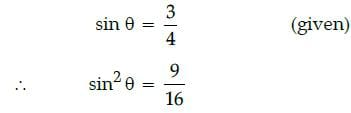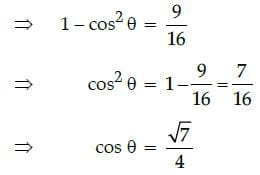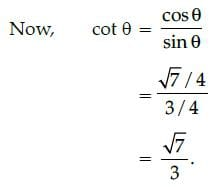Test: Class 10 Mathematics: CBSE Sample Question Paper- Term I (2021-22) - Question 33

If cos θ = 4/5, then tan θ =

Detailed Solution for Test: Class 10 Mathematics: CBSE Sample Question Paper- Term I (2021-22) - Question 33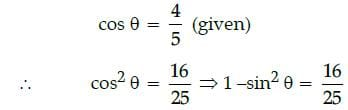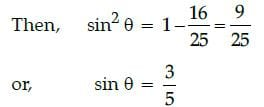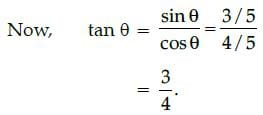Test: Class 10 Mathematics: CBSE Sample Question Paper- Term I (2021-22) - Question 34

If two positive integers p and q are written as p = a3b4 and q = a4b4, where a and b are prime numbers,then LCM (p, q) × HCF (p, q) is :

Detailed Solution for Test: Class 10 Mathematics: CBSE Sample Question Paper- Term I (2021-22) - Question 34

p = a3b4
and q = a4b4
Then LCM (p, q) = a4 b4
and HCF (p, q) = a3 b4
∴ LCM (p, q) x HCF (p, q)
= a4 b4 x a3 b4
= a7 b8

Test: Class 10 Mathematics: CBSE Sample Question Paper- Term I (2021-22) - Question 35

In evening, during family time, Radha observed the dimensions of room. If the length, breadth andheight of a room are 24 m, 18 m and 9 m respectively, then the length of the longest rod that canmeasure the dimensions of the room exactly is :

Detailed Solution for Test: Class 10 Mathematics: CBSE Sample Question Paper- Term I (2021-22) - Question 35

Length = 24 m, breadth = 18 m and height = 9 m
Since, the length of the longest rod is equal to HCF (24, 18, 9), i.e., 24 = 23 × 3
18 = 2 × 32
and 9 = 32
Then, HCF (24, 18 and 9) = 3
Thus, the longest rod that can measure the dimensions of the room exactly = 3 m.

Test: Class 10 Mathematics: CBSE Sample Question Paper- Term I (2021-22) - Question 36

The value of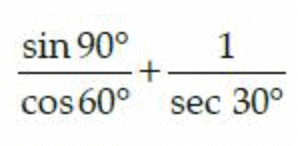is:

Detailed Solution for Test: Class 10 Mathematics: CBSE Sample Question Paper- Term I (2021-22) - Question 36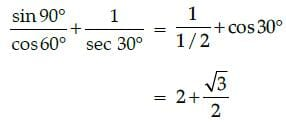Test: Class 10 Mathematics: CBSE Sample Question Paper- Term I (2021-22) - Question 37

If DABC ~ DQRP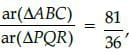and AC = 18 cm then the value of QR is :

Detailed Solution for Test: Class 10 Mathematics: CBSE Sample Question Paper- Term I (2021-22) - Question 37

∵  DABC ~ DQRP, then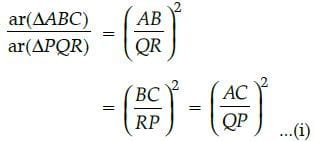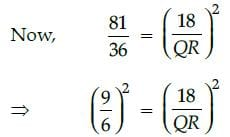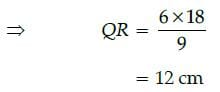Test: Class 10 Mathematics: CBSE Sample Question Paper- Term I (2021-22) - Question 38

Rational number 7/125 will terminate after ........ decimal places.

Detailed Solution for Test: Class 10 Mathematics: CBSE Sample Question Paper- Term I (2021-22) - Question 38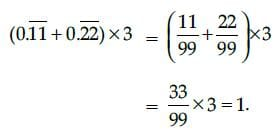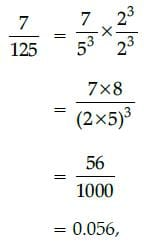which terminates after 3 places.

Test: Class 10 Mathematics: CBSE Sample Question Paper- Term I (2021-22) - Question 39

The difference of (3 + 2 √3) and (3 − 2 √3) is :

Detailed Solution for Test: Class 10 Mathematics: CBSE Sample Question Paper- Term I (2021-22) - Question 39

Difference = (3 + 2√3) −(3 − 2√3)
= 3 + 2√3 − 3 + 2√3
= 4√3 ,
which is irrational.

Test: Class 10 Mathematics: CBSE Sample Question Paper- Term I (2021-22) - Question 40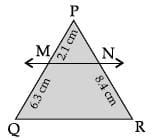In the given figure, MN || QR. The length of PN is :

Detailed Solution for Test: Class 10 Mathematics: CBSE Sample Question Paper- Term I (2021-22) - Question 40

In DPQR, MN || QR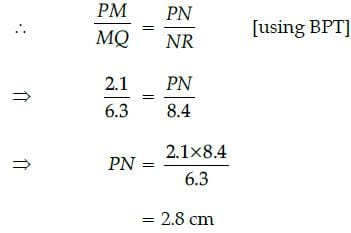Test: Class 10 Mathematics: CBSE Sample Question Paper- Term I (2021-22) - Question 41

Case Study
Places A and B are 80 km apart on a highway. One car starts from A and another from B at the same time. If the cars travel in the same direction at different speeds, they meet in 4 hours. If they travel towards each other, they meet in 1 hour.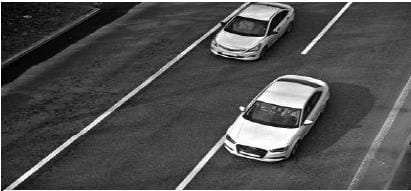Assuming that the speed of first car and second car be u km/h and v km/h respectively.

What is the relative speed of both cars while they are travelling in the same direction ?

Detailed Solution for Test: Class 10 Mathematics: CBSE Sample Question Paper- Term I (2021-22) - Question 41

Relative speed of both cars while they are travelling in same direction
= (50 – 35) km/hr = 15 km/h.

Test: Class 10 Mathematics: CBSE Sample Question Paper- Term I (2021-22) - Question 42

Case Study
Places A and B are 80 km apart on a highway. One car starts from A and another from B at the same time. If the cars travel in the same direction at different speeds, they meet in 4 hours. If they travel towards each other, they meet in 1 hour.What is the relative speed of both cars while they are travelling towards each other ?

Detailed Solution for Test: Class 10 Mathematics: CBSE Sample Question Paper- Term I (2021-22) - Question 42

Relative speed of both cars while they are travelling in opposite directions i.e., travelling towards each other
= (50 + 35) km/hr = 85 km/h.

Test: Class 10 Mathematics: CBSE Sample Question Paper- Term I (2021-22) - Question 43

Case Study
Places A and B are 80 km apart on a highway. One car starts from A and another from B at the same time. If the cars travel in the same direction at different speeds, they meet in 4 hours. If they travel towards each other, they meet in 1 hour.What is the actual speed of one car?

Detailed Solution for Test: Class 10 Mathematics: CBSE Sample Question Paper- Term I (2021-22) - Question 43

Let the speeds of first car and second car be u km/hr and v km/hr respectively.
According to the given information.
4 (u – v) = 80
i.e,. u – v = 20 ...(i)
and u + v = 80 ...(ii)
Solving eqs. (i) and (ii), we get u = 50 km/hr.

Test: Class 10 Mathematics: CBSE Sample Question Paper- Term I (2021-22) - Question 44

Case Study
Places A and B are 80 km apart on a highway. One car starts from A and another from B at the same time. If the cars travel in the same direction at different speeds, they meet in 4 hours. If they travel towards each other, they meet in 1 hour.What is the actual speed of other car?

Detailed Solution for Test: Class 10 Mathematics: CBSE Sample Question Paper- Term I (2021-22) - Question 44

Let the speeds of first car
and second car be u km/hr and v km/hr
respectively.
According to the given information.
4 (u – v) = 80
i.e,. u – v = 20 ...(i)
and u + v = 80 ...(ii)
Solving eqs. (i) and (ii), we get u = 50
km/hr and v = 30 km/hr.

Test: Class 10 Mathematics: CBSE Sample Question Paper- Term I (2021-22) - Question 45

Case Study
Places A and B are 80 km apart on a highway. One car starts from A and another from B at the same time. If the cars travel in the same direction at different speeds, they meet in 4 hours. If they travel towards each other, they meet in 1 hour.The given problem is based on which mathematical concept

Detailed Solution for Test: Class 10 Mathematics: CBSE Sample Question Paper- Term I (2021-22) - Question 45

The given problem is based
on pair of linear equations.

Test: Class 10 Mathematics: CBSE Sample Question Paper- Term I (2021-22) - Question 46

Case Study

The diagram show the plans for a sun room. It will be built onto the wall of a house. The four walls of
the sun room are square clear glass panels. The roof is made using,

• Four clear glass panels, trapezium in shape, all of the same size
• One tinted glass panel, half a regular octagon in shape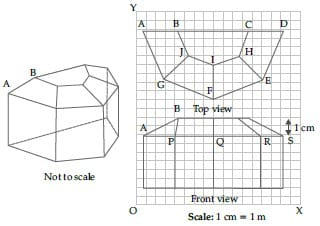Refer to Top View, find the mid-point of the segment joining the points J(6, 17) and I(9, 16).

Detailed Solution for Test: Class 10 Mathematics: CBSE Sample Question Paper- Term I (2021-22) - Question 46

Mid-point of J(6, 17) and I (9, 16) is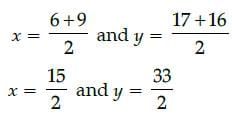Test: Class 10 Mathematics: CBSE Sample Question Paper- Term I (2021-22) - Question 47

Case Study

The diagram show the plans for a sun room. It will be built onto the wall of a house. The four walls of
the sun room are square clear glass panels. The roof is made using,

• Four clear glass panels, trapezium in shape, all of the same size
• One tinted glass panel, half a regular octagon in shapeRefer to front View, the distance of the point P from the y-axis is:

Detailed Solution for Test: Class 10 Mathematics: CBSE Sample Question Paper- Term I (2021-22) - Question 47

The distance of the point P from the Y-axis = 4.

Test: Class 10 Mathematics: CBSE Sample Question Paper- Term I (2021-22) - Question 48

Case Study

The diagram show the plans for a sun room. It will be built onto the wall of a house. The four walls of
the sun room are square clear glass panels. The roof is made using,

• Four clear glass panels, trapezium in shape, all of the same size
• One tinted glass panel, half a regular octagon in shapeRefer to front view, the distance between the points A and S is

Detailed Solution for Test: Class 10 Mathematics: CBSE Sample Question Paper- Term I (2021-22) - Question 48

A's coordinates = (1, 8)
S's coordinates = (15, 8)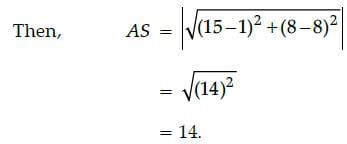Test: Class 10 Mathematics: CBSE Sample Question Paper- Term I (2021-22) - Question 49

Case Study

The diagram show the plans for a sun room. It will be built onto the wall of a house. The four walls of
the sun room are square clear glass panels. The roof is made using,

• Four clear glass panels, trapezium in shape, all of the same size
• One tinted glass panel, half a regular octagon in shapeRefer to front view, find the co-ordinates of the point which divides the line segment joining the points
A and B in the ratio 1 : 3 internally.

Detailed Solution for Test: Class 10 Mathematics: CBSE Sample Question Paper- Term I (2021-22) - Question 49

The coordinates of A = (1, 8)
The coordinates of B = (4, 10)
Also, m = 1 and n = 3
Then,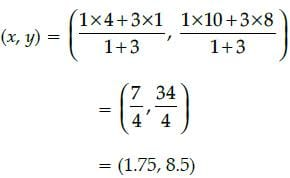Test: Class 10 Mathematics: CBSE Sample Question Paper- Term I (2021-22) - Question 50

Case Study

The diagram show the plans for a sun room. It will be built onto the wall of a house. The four walls of
the sun room are square clear glass panels. The roof is made using,

• Four clear glass panels, trapezium in shape, all of the same size
• One tinted glass panel, half a regular octagon in shapeRefer to front view, if a point (x, y) is equidistant from the Q(9, 8) and S(17, 8), then

Detailed Solution for Test: Class 10 Mathematics: CBSE Sample Question Paper- Term I (2021-22) - Question 50

Let point be P(x, y)
PQ2 = PS2
or, (x – 9)2 + (y – 8)2 = (x – 17)2 + (y – 8)2
or, x – 13 = 0

## CBSE Sample Papers For Class 10

174 docs|7 tests
 Use Code STAYHOME200 and get INR 200 additional OFF Use Coupon Code
Information about Test: Class 10 Mathematics: CBSE Sample Question Paper- Term I (2021-22) Page
In this test you can find the Exam questions for Test: Class 10 Mathematics: CBSE Sample Question Paper- Term I (2021-22) solved & explained in the simplest way possible. Besides giving Questions and answers for Test: Class 10 Mathematics: CBSE Sample Question Paper- Term I (2021-22), EduRev gives you an ample number of Online tests for practice

174 docs|7 tests